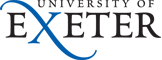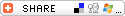Module Description
PHY2025 Mathematics with Physical Applications 2013-14
Dr F.Y. Ogrin

Delivery Weeks: T1:01-11, T2:01-11
Level: 5 (NQF)
Credits: 15 NICATS / 7.5 ECTS
Enrolment: 71 students (approx)

### Description

This module will enable the student to build on the knowledge and skills developed in PHY1026 in order to achieve a deeper understanding of and greater competence in some central mathematical ideas and techniques used throughout physics. The emphasis is on practical skills rather than formal proofs. Students will acquire skills in some key techniques that relate directly to the advanced modules they will meet in the later stages of their degree programme, but also have wide applicability across the mathematical sciences.

### Module Aims

This module pre-dates the current template; refer to the description above and the following ILO sections.

### Intended Learning Outcomes (ILOs)

A student who has passed this module should be able to:

• Module Specific Skills and Knowledge:
1. use probability theory to solve problems;
2. calculate Fourier transforms and use them to solve problems
3. solve partial differential equations by separation of variables;
4. calculate eignvalues and eigenvectors and apply the the techniques to physical problems;
5. use basis vectors to transform differential operator equations to matrix form and hence apply eigen equation techniques;
6. obtain approximate solutions to differential equations through the use of perturbation theory;
• Discipline Specific Skills and Knowledge:
1. apply analytical and numerical skills in mathematics;
• Personal and Key Transferable / Employment Skills and Knowledge:
1. formulate and tackle problems in a logical and systematic manner;
2. present work clearly with justification of techniques and methods;
3. work co-operatively with peers and with the demonstrators to solve guided problems.

### Syllabus Plan

1. Probability theory
1. Random variables
2. Conditional probability
3. Probability distributions
1. Discrete
2. Continuous
2. The Dirac delta-function
3. Fourier transforms including the convolution theorem
4. Solution of linear partial differential equations
1. Simple second order differential equations and common varieties: Harmonic oscillator, Schrödinger equation, Poisson's equation, wave equation and diffusion equation.
2. Separation of variables: The Laplacian family of equations in physics, separation of variables, mechanics of the technique, form of solutions, general solutions in series form, relation to Fourier series, spatial boundary conditions, time dependence, initial conditions.
3. Examples: rectangular drum, classical and quantum harmonic oscillator, waves at a boundary, temperature distributions, wavepacket/quantum particle in a box
4. Role of symmetry: Cylindrical and spherical polar co-ordinates, appearance of special functions. Use of special functions by analogy to sin, cos, sinh, cosh etc.
5. Examples: circular drum, hydrogen wave function
5. Linear Algebra
1. Revision: Row and column vectors, matrices, matrix algebra, the solutions of systems of linear equations.
2. Eigenvalue equations I: The matrix equation Ax=ax, solving the matrix equation, the secular determinant, eigenvalues and eigenvectors, canonical form, normal modes/harmonics, simple coupled oscillators.
3. Eigenvalue equations II: Properties of eigenvectors: orthogonality, degeneracy, as basis vectors.
4. Eigenvalue equations III: Differential equations as eigenvalue equations and the matrix representation Ax=ax; choosing the basis, solving the equation, the secular determinant, eigenvalues and eigenvectors.
5. Examples: classical coupled modes, Schrödinger wave equation
6. Approximate solutions to differential equations (perturbation theory): use of eigenvectors, first- and second-order through repeated substitution, problem of degeneracies.
7. Examples: quantum particle in a well, a mass on drum, coupled particles

### Learning and Teaching

#### Learning Activities and Teaching Methods

Description Study time KIS type
22×1-hour lectures 22 hours SLT
8×2-hour self-study packages 16 hours GIS
10×3-hour problems sets 30 hours GIS
Problems class support 11 hours SLT
Tutorial support 3 hours SLT
Reading, private study and revision 68 hours GIS

#### Assessment

Weight Form Size When ILOS assessed Feedback
0% Exercises set by tutor 6×30-minute sets (typical) Scheduled by tutor 1-10 Discussion in tutorials
0% Guided self-study 8×2-hour packages Fortnightly 1-10 Discussion in tutorials
20% 10 × Problems Sets 3 hours per set Fortnightly 1-10 Marked in problems class, then discussed in tutorials
15% Mid-term Test 1 30 minutes Weeks T1:06 1-9 Marked, then discussed in tutorials
15% Mid-term Test 2 30 minutes Weeks T2:06 1-9 Marked, then discussed in tutorials
50% Final Examination 120 minutes May/June assessment period 1-9 Mark via MyExeter, collective feedback via ELE and solutions.

### Resources

The following list is offered as an indication of the type & level of information that students are expected to consult. Further guidance will be provided by the Module Instructor(s).

Core text:

Supplementary texts:

ELE:

### Further Information

#### Prior Knowledge Requirements

Pre-requisite Modules Mathematics for Physicists (PHY1026) none

#### Re-assessment

Re-assessment is not available except when required by referral or deferral.

Original form of assessment Form of re-assessment ILOs re-assessed Time scale for re-assessment
Whole module Written examination (100%) 1-9 August/September assessment period

Notes: See Physics Assessment Conventions.

#### KIS Data Summary

Learning activities and teaching methods
SLT - scheduled learning & teaching activities 36 hrs
GIS - guided independent study 114 hrs
PLS - placement/study abroad 0 hrs
Total 150 hrs
Summative assessment
Coursework 20%
Written exams 80%
Practical exams 0%
Total 100%

#### Miscellaneous

 IoP Accreditation Checklist MT-07 Solution of linear partial differential equations MT-12 Fourier series and transforms including the convolution theorem. MT-13 Probability distributions. Availability unrestricted Distance learning NO Keywords Physics; Equation; Differentiation; Eigenvalue; Solution; Eigenvectors; Matrix; Probabilities; Wave; Variables; Differential equations. Created 01-Oct-10 Revised 01-Oct-11
 Validate   Link-check © Copyright & disclaimer